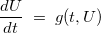# Time schemes

Several time schemes have been implemented, we distinguish explicit time-schemes from implicit time-schemes. You can use the implementation of time schemes provided by Montjoie for your own evolution problem. The model problem is the first-order evolution problem :An example of use of time schemes for this equation is detailed below

```class MyFunction
{
public :
void EvaluateFunction(const Real_wp& t, VectReal_wp& u, VectReal_wp& gU)
{
// you fill g(t, U) in vector gU
gU(0) = u(1);
gU(1) = -u(0);
}
};

// instance of MyFunction
MyFunction sys;

// for example we consider Runge-Kutta explicit scheme
RungeKutta_Iterator<Real_wp> RK;

// initialization of coefficients by giving the order
RK.SetOrder(4);

// initialization of the scheme by providing the first iterate and time step
Real_wp t0 = 0, dt = 0.01;
VectReal_wp U0(N);
U0.Fill(0);
RK.SetInitialCondition(t0, dt, U0, sys);

// then loop in times
for (int nt = 0; nt < nb_max_iterations; nt++)
{
// computation of U^n+1
// the result is present in RK.Y
}

// you can release memory used by the time scheme (intermediary vectors)
RK.Clear();
```

### Time schemes for first-order evolution systems

 RungeKutta_Iterator explicit Runge-Kutta schemes Talezer_Iterator explicit Tal-ezer schemes AdamsBashforth_Moulton_Iterator explicit Adams-Bashforth-Moulton schemes TaylorSeries_Iterator explicit scheme based on Taylor expansion LowStorageRK_Iterator explicit low-storage Runge-Kutta schemes MultiStepButcher_Iterator explicit multistep Butcher's schemes OptimalModifiedEquation_Iterator optimal modified equation schemes SdirkScheme_Iterator Singly-diagonally implicit Runge-Kutta schemes SdirkScheme_Iterator Singly-diagonally implicit Runge-Kutta schemes LocalImperialeScheme_Iterator local-time stepping with optimal modified equation approach (Imperiale's scheme) LocalPipernoScheme_Iterator local-time stepping with symplectic approach (Piperno's scheme) ModifiedEquationSystemIterator modified equation approach

### Time schemes for second-order evolution systems

 TetaScheme_Iterator implicit theta-scheme ModifiedEquationIterator modified equation approach

### Functions related to unsteady simulations

 RunTimeScheme completes time simulation of a first-order evolution system RunFirstOrderScheme completes time simulation of a first-order evolution system RunSecondOrderScheme completes time simulation of a second-order evolution system ExtractSubMesh extraction of a small mesh for the computation of the local time step EvaluateCFL evaluation of the global CFL number ComputeLocalTimeStep computation of the local CFL for each element of the mesh

### Functions related to unsteady simulations

 RunTimeScheme completes time simulation of a first-order evolution system

### Public methods of VarInstationary

 GetNbDof returns the size of the evolution system GetNormeSolution returns the L2 norm of the solution WriteSnapshot writes outputs (if needed) at a given time FirstOrderScheme returns true if the evolution system involves only first-order derivatives ImplicitScheme returns true if the selected scheme is implicit SetTimeScheme sets the time scheme to use InitTimeIterations initialisation beforing running time iterations GetCflScheme returns the coefficient involved in stability condition of the selected time scheme ComputeStiffnessMatrix computes stiffness matrix ComputeMassMatrix computes mass and damping matrix GiveLevelTime initialization of time levels for local time-stepping SetLevel sets level (which part of stiffness matrix will be involved) GetNumberOfUnknowns computes and returns the size of the evolution system GetNbScalarUnknowns returns the number of degrees of freedom for the scalar unknown GetNbVectorialUnknowns returns the number of degrees of freedom for the vectorial unknown GetNbVectorialUnknownsPML returns the number of degrees of freedom for the vectorial unknown and for PML elements RunTimeIterations runs time iterations RunAll runs complete simulation (from reading input file until writing snapshots at regular time intervals) EvaluateFunction evaluation of g(t,U) in the evolution system du/dt = g(t, U) EvaluateDerivativeFunction evaluation of time derivatives of g(t,U) in the evolution system du/dt = g(t, U) ApplyOperatorKh multiplication by stiffness matrix ApplyOperatorDh multiplication by scalar mass matrix ApplyOperatorSh multiplication by scalar damping matrix ApplyOperatorShVectorial multiplication by vectorial damping matrix ApplyOperatorDhMinusdtSh multiplication by matrix Dh - Δ t/2 Sh SolveOperatorDhPlusdtSh resolution by matrix Dh + Δ t/2 Sh SolveOperatorDh resolution by scalar mass matrix SolveCholeskyDh resolution of system L x = y or LT x = y where Dh = L LT SolveOperatorDhPlusGammaKh resolution by matrix Dh + γ Kh ApplyOperatorRhScalar multiplication by scalar stiffness matrix ApplyOperatorRhVectorial multiplication by vectorial stiffness matrix ApplyOperatorBhMinusdtSh multiplication by matrix Bh - Δ t/2 Sh SolveOperatorBhPlusdtSh resolution by matrix Bh + Δ t/2 Sh SolveOperatorBh resolution by vectorial mass matrix SolveMassMatrix resolution by mass matrix Assemble assembles global vector (for parallel computations) SetInitialVector computation of the initial condition GiveIterate method called in RunTimeScheme to provide an iterate GiveVectorialIterate method called in RunFirstOrderScheme to provide the vectorial iterate GiveFinalIterate method called in RunTimeScheme to provide the final iterate GiveNumberIterations method called in RunTimeScheme to provide the number of iterations